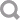eBay Post-Order APIVersion 2.6# Amount

This type is used to expressed dollar amounts and the currency used.

Types that use Amount:

Calls that use Amount:

## Fields

convertedFromCurrency ( CurrencyCodeEnum ) [0..?]
Gets the original transaction currency, if the transaction involves a currency conversion. The currency is represented as a 3-letter currency code from ISO4217. See http://en.wikipedia.org/wiki/ISO_4217 If no conversion occurs, this should not be populated.
See the Field Index to learn which calls use convertedFromCurrency.

convertedFromValue ( number ) [0..?]
Gets the original transaction amount, if the transaction involves a currency conversion. If no conversion occurs, this should not be populated.
See the Field Index to learn which calls use convertedFromValue.

currency ( CurrencyCodeEnum ) [0..?]
Gets the transaction currency. This is the currency in which the transaction occurs: in the case of currency conversion, this is the post-conversion currency.
See the Field Index to learn which calls use currency.

value ( number ) [0..?]
Gets the amount of the transaction, expressed in the specified request.
See the Field Index to learn which calls use value.